## 最简单的写表格

``````@Data
public class Item {
@ExcelProperty("字符串标题")
private String string;
@ExcelProperty("日期标题")
private Date date;
@ExcelProperty("数字标题")
private Double doubleData;
@ExcelIgnore
private String ignore;
}``````

### 根据头对象和列表向一个工作表中写一个表格

``````/**
* <p>
* 提供列表和函数作为数据源
*/
public static void writeAutoWriter() {
final String fileName = defaultFileName("writeAutoWriter");
EasyExcelFactory.write(fileName)
.sheet("模板")
.doWrite(WriteSample::sampleItems);
}``````

EasyExcel提供了`EasyExcelFactory`类，API方法也是fluent方式，可以如丝般顺滑的实现生成Excel文件。如果感觉`EasyExcelFactory`太长，还可以直接写作`EasyExcel`，这是`EasyExcelFactory`的子类，类似于别名。

`com.alibaba.excel.EasyExcelFactory#write(java.lang.String)`方法的参数传的是导出文件的文件名，如果并不需要生成文件，只需要创建文件流，也可以传入一个输出流`OutputStream`，这样就可以更加灵活的实现生成逻辑了。

`com.alibaba.excel.metadata.AbstractParameterBuilder#head()`方法是定义表头，只要传入一个类，就会读取这个类的所有字段作为表头。如果字段上`com.alibaba.excel.annotation.ExcelProperty`注解，定义了`value`，就会取`value`的值作为表头。此处还有很多操作，比如，`value`是数组，可以定义多个，如果是相邻字段定义了相同的表头，会合并单元格，表体内容会选择第一个单元格的内容。这个注解还可以定义`index``order``converter`等，后面会一一给出例子。

`com.alibaba.excel.write.builder.ExcelWriterBuilder#sheet()`方法定义工作表，有多个重载方法，可以定义`sheetNo`指明是第几个工作表，可以传入`sheetName`指明工作表名称。

`com.alibaba.excel.write.builder.ExcelWriterSheetBuilder#doWrite()`方法就是写Excel文件了，传入全部的列表数据，或者使用Java8+的`Supplier`函数。还可以实现分页写入，后面会给出例子。这个方法会自动关闭文件流，真是很贴心。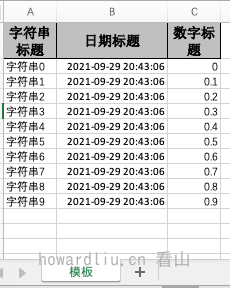### 根据头对象和列表向多个工作表中写数据

``````/**
* <p>
* 提供列表和函数作为数据源
*/
public static void writeManualWither() {
String fileName = defaultFileName("writeManualWriter");
ExcelWriter excelWriter = null;
try {
excelWriter = EasyExcelFactory.write(fileName)
.build();
final WriteSheet writeSheet1 = EasyExcelFactory.writerSheet("模板1").build();
excelWriter.write(WriteSample::sampleItems, writeSheet1);

final WriteSheet writeSheet2 = EasyExcelFactory.writerSheet("模板2").build();
excelWriter.write(sampleItems(), writeSheet2);
} finally {
// 千万别忘记finish 会帮忙关闭流
if (excelWriter != null) {
excelWriter.finish();
}
}
}``````

`com.alibaba.excel.ExcelWriter`类是Excel写对象，用来创建Excel工作簿的。`com.alibaba.excel.write.metadata.WriteSheet`类是Sheet写对象，用来创建Sheet工作表的。通过`com.alibaba.excel.ExcelWriter#write()`方法，指定写入数据和写入的目标工作表，就可以实现向多个工作表中写数据的功能。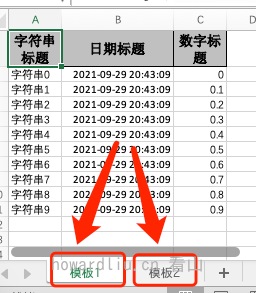## 按照定义指定导出列

### 排除指定列

``````private static void writeExcludeColumn() {
String fileName = defaultFileName("writeExcludeColumn");
Set<String> excludeColumnFiledNames = new HashSet<>();

EasyExcelFactory.write(fileName)
.excludeColumnFiledNames(excludeColumnFiledNames)
.sheet("模板")
.doWrite(WriteSample::sampleItems);
}``````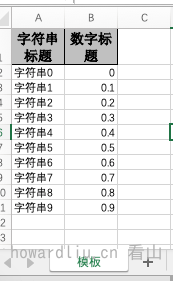### 只导出指定列

``````private static void writeIncludeColumn() {
String fileName = defaultFileName("writeIncludeColumn");
Set<String> includeColumnFiledNames = new HashSet<>();
EasyExcelFactory.write(fileName)
.includeColumnFiledNames(includeColumnFiledNames)
.sheet("模板")
.doWrite(WriteSample::sampleItems);
}``````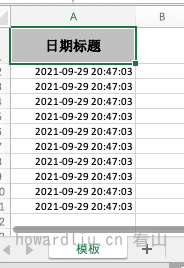## 表头

### 原始表头

``````@Data
public class EmptyItem {
private String string;
private Date date;
private Double doubleData;
}``````

``````private static void writeNoAnnotation() {
final String fileName = defaultFileName("writeNoAnnotation");
EasyExcelFactory.write(fileName)
.sheet("模板")
.doWrite(WriteSample::sampleItems);
}``````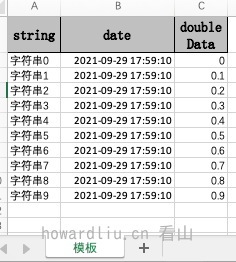### 自定义表头

``````@Data
public class IndexItem {
@ExcelProperty(value = "字符串标题", index = 1)
private String string;
@ExcelProperty(value = "日期标题", index = 3)
private Date date;
@ExcelProperty(value = "数字标题", index = 5)
private Double doubleData;
}``````

``````private static void writeWithIndex() {
final String fileName = defaultFileName("writeWithIndex");
EasyExcelFactory.write(fileName)
.sheet("模板")
.doWrite(WriteSample::sampleItems);
}``````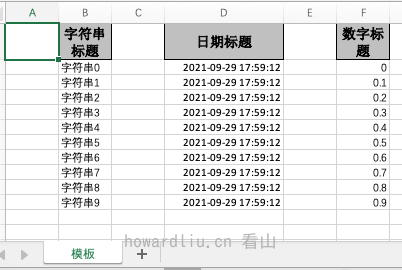`ExcelProperty`的value属性是字符串数组，相当于一个字段可以定义多个头，这样就可以实现多级表头。同时，如果位置相邻的列定义列明相同，还会合并列。比如：

``````@Data
@ExcelProperty({"大标题", "字符串标题"})
private String string;
@ExcelProperty("日期标题")
private Date date;
@ExcelProperty({"大标题", "数字标题0"})
private Double doubleData;
@ExcelProperty({"数字标题"})
private Double doubleData1;
@ExcelProperty({"数字标题"})
private Double doubleData2 = 0.0;
}``````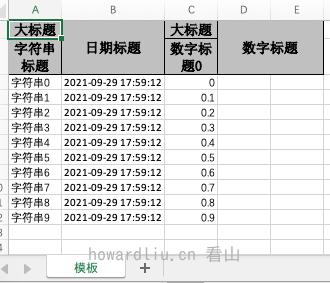### 动态表头、表体

``````/**
* 动态表头，传入的是{@code List<List<String>>}格式数据。
* <p>
* 可以实现多层表头。
*/
EasyExcelFactory.write(fileName)
.sheet()
.doWrite(sampleItems());
}

final List<String> head0 = new ArrayList<>(Arrays.asList("头0", "字符串标题【动态】"));
final List<String> head1 = new ArrayList<>(Arrays.asList("头0", "日期标题【动态】"));
final List<String> head2 = new ArrayList<>(Collections.singletonList("数字标题【动态】"));
}``````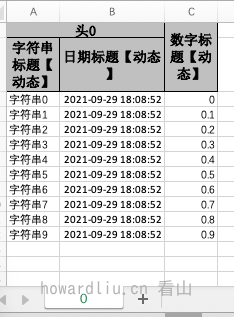``````private static void writeDynamicData() {
String fileName = defaultFileName("writeDynamicData");
EasyExcelFactory.write(fileName)
.sheet()
.doWrite(dynamicData());
}

private static List<List<Object>> dynamicData() {
List<List<Object>> list = new ArrayList<>();
for (int i = 0; i < 10; i++) {
List<Object> data = new ArrayList<>();
}
return list;
}``````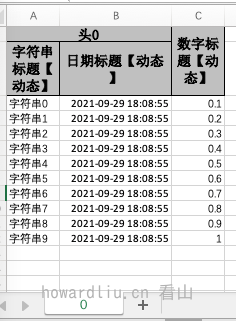### 表头国际化

``````@Data
public class FormatContentItem {
@ExcelProperty(value = "字符串标题", converter = TitleFormatConverter.class)
private String string;
@DateTimeFormat("yyyy年MM月dd日HH时mm分ss秒")
@ExcelProperty(value = "日期标题")
private Date date;
@NumberFormat("0.000%")
@ExcelProperty("数字标题")
private Double doubleData;
}``````

``````/**
* <p>
*/
EasyExcelFactory.write(fileName)
.sheet()
.doWrite(sampleItems());
}``````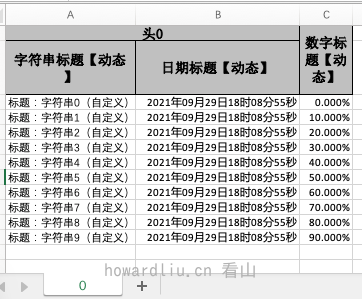## 多次写入

### 向同一个excel同一个sheet中多次写入

``````private static void writeOneSheet() {
String fileName = defaultFileName("writeOneSheet");
ExcelWriter excelWriter = null;
try {
excelWriter = EasyExcelFactory.write(fileName)
.build();
final WriteSheet writeSheet = EasyExcelFactory.writerSheet("模板").build();
for (int i = 0; i < 5; i++) {
excelWriter.write(sampleItems(), writeSheet);
}
} finally {
// 千万别忘记finish 会帮忙关闭流
if (excelWriter != null) {
excelWriter.finish();
}
}
}``````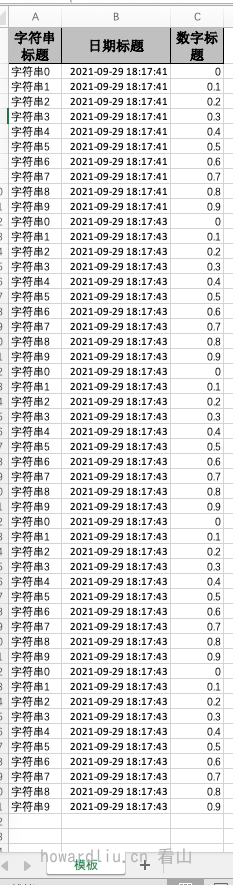### 向同一个excel不同sheet中多次写入（表头相同）

``````private static void writeDiffSheetWithSameHead() {
ExcelWriter excelWriter = null;
try {
excelWriter = EasyExcelFactory.write(fileName)
.build();
for (int i = 0; i < 5; i++) {
final WriteSheet writeSheet = EasyExcelFactory.writerSheet(i, "模板" + i)
.build();
excelWriter.write(sampleItems(), writeSheet);
}
} finally {
// 千万别忘记finish 会帮忙关闭流
if (excelWriter != null) {
excelWriter.finish();
}
}
}``````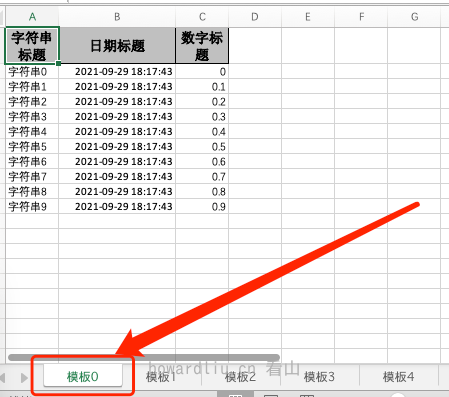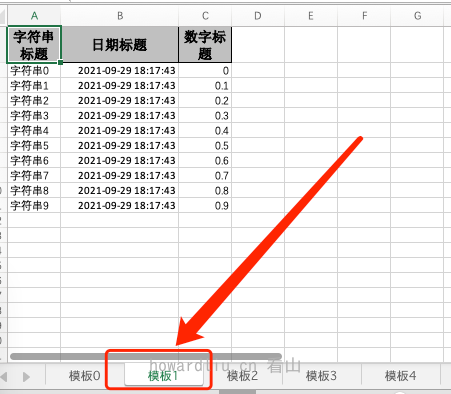### 向同一个excel不同sheet中多次写入（表头不同）

``````private static void writeDiffSheetWithDiffHead() {
ExcelWriter excelWriter = null;
try {
excelWriter = EasyExcelFactory.write(fileName)
.build();

final WriteSheet writeSheet0 = EasyExcelFactory.writerSheet(0, "模板1")
.build();
excelWriter.write(sampleItems(), writeSheet0);

final WriteSheet writeSheet1 = EasyExcelFactory.writerSheet(1, "模板2")
.build();
excelWriter.write(sampleItems(), writeSheet1);
} finally {
// 千万别忘记finish 会帮忙关闭流
if (excelWriter != null) {
excelWriter.finish();
}
}
}``````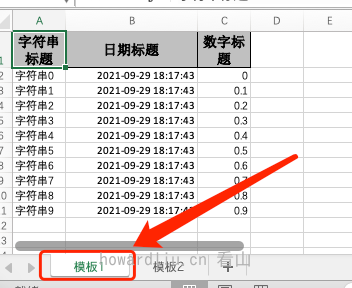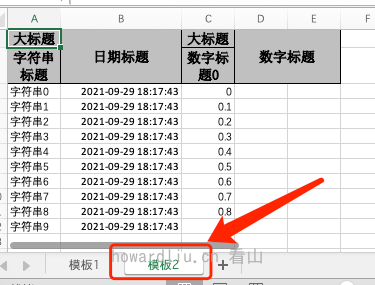## 实现多表

EasyExcel对于Excel文件定义分成了三层，

1. 工作簿，也就是Excel文件
2. 工作表，对应是Excel文件中的Sheet
3. 表格，对应是Sheet中的有表头、表体的组合

### 同一表单中创建表格

``````private static void writeTable() {
String fileName = defaultFileName("writeTable");
final ExcelWriter excelWriter = EasyExcelFactory.write(fileName)
.build();
try {
// 把sheet设置为不需要头 不然会输出sheet的头 这样看起来第一个table 就有2个头了
WriteSheet writeSheet = EasyExcelFactory.writerSheet()
.build();

// 这里必须指定需要头，table 会继承sheet的配置，sheet配置了不需要，table 默认也是不需要
WriteTable writeTable0 = EasyExcelFactory.writerTable(0)
.build();

excelWriter.write(sampleItems(), writeSheet, writeTable0);
} finally {
if (excelWriter != null) {
excelWriter.finish();
}
}
}``````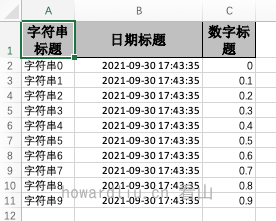### 同一表单中创建不同表格（相同表头）

``````private static void writeTables() {
String fileName = defaultFileName("writeTables");
final ExcelWriter excelWriter = EasyExcelFactory.write(fileName)
.build();
try {
// 把sheet设置为不需要头 不然会输出sheet的头 这样看起来第一个table 就有2个头了
WriteSheet writeSheet = EasyExcelFactory.writerSheet()
.build();

// 这里必须指定需要头，table 会继承sheet的配置，sheet配置了不需要，table 默认也是不需要
WriteTable writeTable0 = EasyExcelFactory.writerTable(0)
.build();

WriteTable writeTable1 = EasyExcelFactory.writerTable(1)
.build();
// 第一次写入会创建头
excelWriter.write(sampleItems(), writeSheet, writeTable0);
// 第二次写如也会创建头，然后在第一次的后面写入数据
excelWriter.write(sampleItems(), writeSheet, writeTable1);
} finally {
if (excelWriter != null) {
excelWriter.finish();
}
}
}``````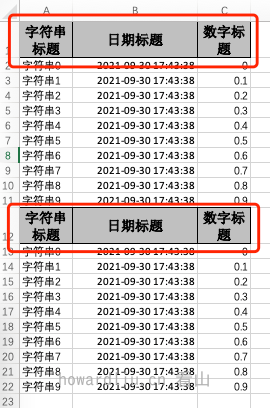### 同一表单中创建不同表格（不同表头）

``````private static void writeTablesWithDiffHead() {
final ExcelWriter excelWriter = EasyExcelFactory.write(fileName)
.build();
try {
WriteSheet writeSheet = EasyExcelFactory.writerSheet()
.build();

WriteTable writeTable0 = EasyExcelFactory.writerTable(0)
.build();
excelWriter.write(sampleItems(), writeSheet, writeTable0);

WriteTable writeTable1 = EasyExcelFactory.writerTable(1)
.build();
excelWriter.write(sampleItems(), writeSheet, writeTable1);
} finally {
if (excelWriter != null) {
excelWriter.finish();
}
}
}``````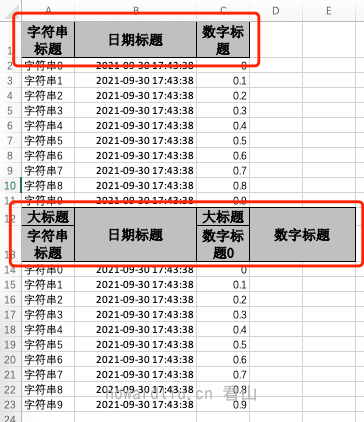## 写入模板文件（非填充）

``````private static void writeByTemplate() {
String fileName = defaultFileName("writeByTemplate");
String templateFile = getPath() + File.separator + "template_write_after_fill.xlsx";
EasyExcelFactory.write(fileName)
.withTemplate(templateFile)
.sheet()
.doWrite(sampleItems());
}``````

1. 指定模板文件路径`ExcelWriterBuilder#withTemplate(java.lang.String)`
2. 指定模板文件对象`ExcelWriterBuilder#withTemplate(java.io.File)`
3. 指定模板文件输入流`ExcelWriterBuilder#withTemplate(java.io.InputStream)`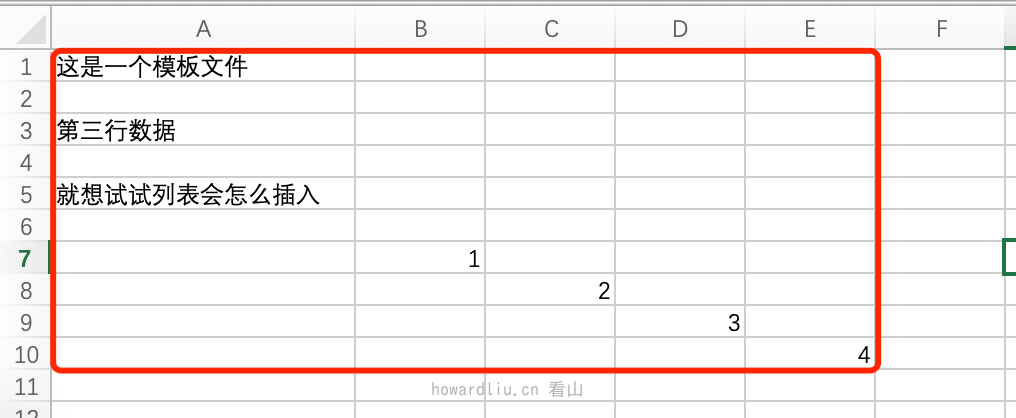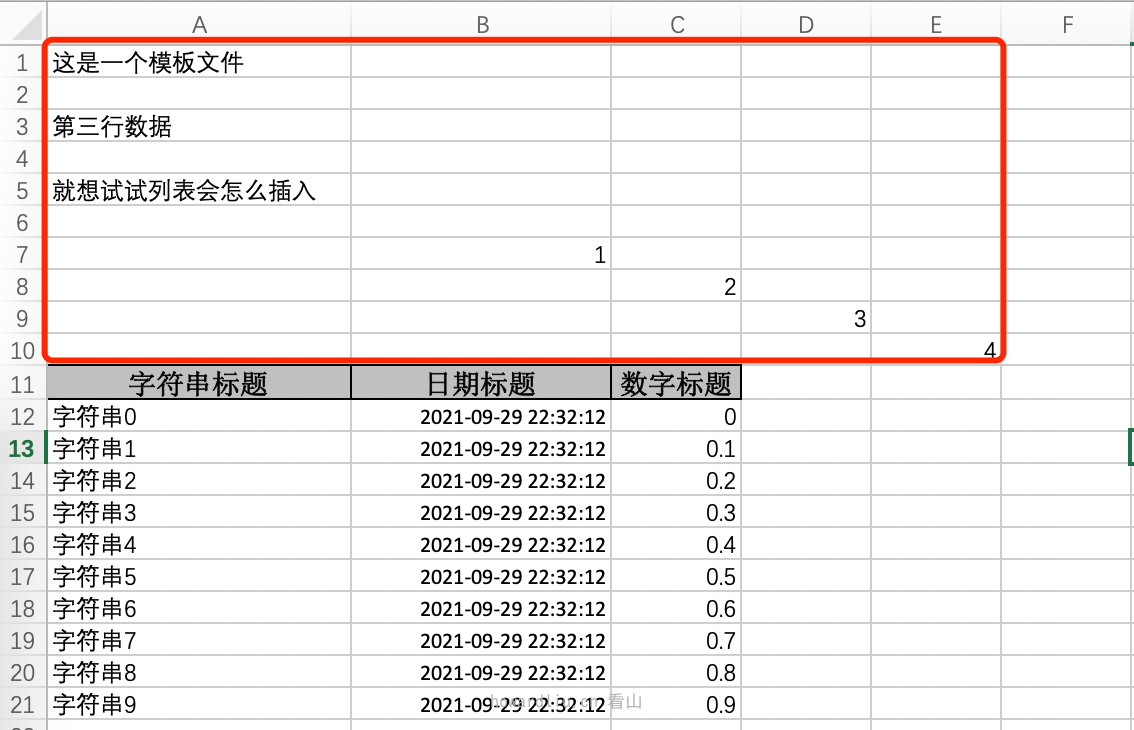## 推荐阅读

👇🏻欢迎关注我的公众号「看山的小屋」，领取精选资料👇🏻